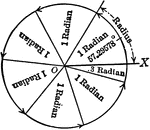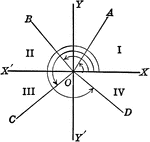Axes showing trigonometric angles, and quadrants.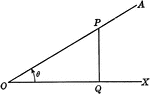### Acute Angle Theta

Illustration of acute angle theta as part of a right triangle.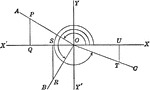### Reference Angles/Triangles Formed by Angles in Quadrants

Lines drawn to horizontal to form triangle ratios (reference triangles, angles).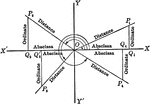### Reference Angles/Triangles Formed by Angles in Quadrants With Labels

Lines drawn to horizontal to form triangle ratios (reference triangles, angles). Axes, quadrants, abscissa,…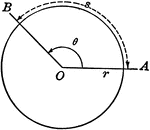### Model of Arc Length, Angle Measure, and Radius

Illustration showing that the arc length can be found by multiplying the angle measure by the radius…